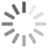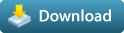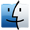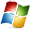# nSoupFrac Voronoi Fracture 1.0.0 for Maya (maya script)

Voronoi Fracture on selection GeomRating: 4.7
(3)

• 2016

#### Operating Systems

•••fracture

per page
1-2 of 2

## Reviews Love it? Or maybe you want to share some creative ways you're putting this item to use.

•#### tankut said over 6 years ago:

Much faster than doing it by hand in Soup - thanks! Made a minor mod to it, to control crack seperation width, here it is: -------------------------------------------------------------------------------------------- #nSoupFrac Voronoi Fracture (with seperation control mod) # #(c) Natapon Huangsakuncharoen, 2015 # #DESCRIPTION: #-Voronoi Fracture on selection Geom # #DISCLAIMER: #-For this script to work you will need the SOuP plug-in by Peter Shipkov # #-Can download from here https://www.soup-dev.com/ # #-The tool is "as is". you can use it for free & at your own risk. # # import maya.cmds as cmds cmds.loadPlugin('SOuP', qt=True) #setRendererInModelPanel base_OpenGL_Renderer modelPanel4; class nSoupFracture(): def __init__(self): if cmds.window("nSoupFrac", exists=1): cmds.deleteUI("nSoupFrac") cmds.window("nSoupFrac", t="nSoupFrac Voronoi Fracture", w=320, h=180) cmds.columnLayout(w=320, h=140) cmds.rowColumnLayout(w=320) cmds.separator( height=10, style='in') cmds.rowColumnLayout(w=320) cmds.text(l="Please select fracture geom") cmds.text(l="") cmds.rowColumnLayout(nc=2,cal=[(1,"right")], cw=[(1,185),(2,135)]) cmds.text('maxNumber', l="Fracture amount ") cmds.intFieldGrp("totalAmount", nf=1, v1=20, cw1=50) cmds.text('seperation', l="Crack width ratio/1000") cmds.intFieldGrp("crackDistance", nf=1, v1=0, cw1=50) cmds.rowColumnLayout(w=320) cmds.button(command=FracIT, l="Fracture me", w=310, al="right", bgc=[0.7,0,0]) cmds.showWindow("nSoupFrac") def FracIT(self, *args): selectGEOMall = cmds.ls(sl=True, type="transform") for selectGEOMone in selectGEOMall: cmds.select(selectGEOMone) #SelectObj Original# selectGEOMplaceDup = cmds.duplicate(rr=True, n="GEOMplaceDup") cmds.makeIdentity (apply=True, t=1, r=1, s=1, n=0, pn=1) cmds.DeleteHistory() cmds.pickWalk (d="down") selectGEOMplaceDupShape = cmds.ls(sl=True, type="mesh") #Create Soup scatter node# soup().create('scatter') cmds.pickWalk (d="down") selectSCATTERshape = cmds.ls(sl=True, type="scatter") cmds.setAttr(selectSCATTERshape + ".scatterMode", 0) cmds.setAttr(selectSCATTERshape + ".pointDensity", 90000) cmds.setAttr(selectSCATTERshape + ".maxNumberOfPoints", 5) #Read Crack Distance FracDistance = .001 * cmds.intFieldGrp("crackDistance",query=True, value1=True) #Create Soup shatter node# soup().create('shatter') selectSHATTERshape = cmds.ls(sl=True, type="shatter") cmds.setAttr(selectSHATTERshape + ".autoEvaluate", 1) cmds.setAttr(selectSHATTERshape + ".distance", FracDistance) #Out put Obj# selectGEOMdup = cmds.polyCube (n="GeomFractureTop", w=1, h=1, d=1, sx=1, sy=1, sz=1, ax=[0,1,0], cuv=4, ch=1) cmds.select(selectGEOMdup) cmds.DeleteHistory() selectGEOMClean = cmds.ls(sl=True, type="transform") cmds.pickWalk (d="down") selectGEOMdupShape = cmds.ls(sl=True, type="mesh") cmds.connectAttr(selectSHATTERshape + ".outGeometry", selectGEOMdupShape + ".inMesh", f=True) #Amount of Fracture pieces# FracAmount = cmds.intFieldGrp("totalAmount",query=True, value1=True) cmds.setAttr(selectSCATTERshape + ".maxNumberOfPoints", FracAmount) #CleanUp out put Obj# cmds.select(selectGEOMClean) cmds.DeleteHistory() cmds.polySeparate (selectGEOMClean, ch=1, n="nGeomFractureTemp") selectGEOMFracture = cmds.ls(sl=True, type="transform") cmds.DeleteHistory(selectGEOMFracture) cmds.CenterPivot(selectGEOMFracture) for FracGeomm in selectGEOMFracture: cmds.select(FracGeomm) FracD = cmds.ls(sl=True, type="transform") cmds.move(rpr=True) selectGEOMmoveX = cmds.getAttr(FracD + ".tx")* -1 selectGEOMmoveY = cmds.getAttr(FracD + ".ty")* -1 selectGEOMmoveZ = cmds.getAttr(FracD + ".tz")* -1 cmds.makeIdentity (apply=True, t=1, r=1, s=1, n=0, pn=1) cmds.setAttr(FracD + ".tx", selectGEOMmoveX) cmds.setAttr(FracD + ".ty", selectGEOMmoveY) cmds.setAttr(FracD + ".tz", selectGEOMmoveZ) cmds.DeleteHistory() for FracGeomRE in selectGEOMFracture: cmds.rename(FracGeomRE, "nGeomFracture") cmds.delete(selectGEOMplaceDup) cmds.select(cl=True) instanceUI = nSoupFracture()
•#### Raj100 said over 6 years ago:

Works great- thanks very much for sharing. Is there a way to control shape of fracture ?

Rate this item: